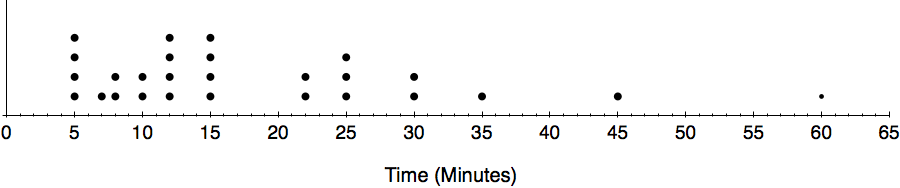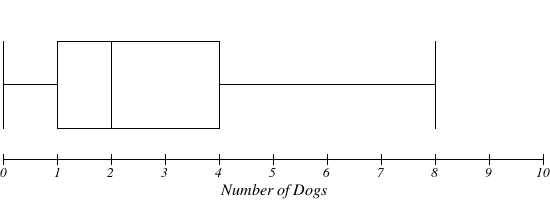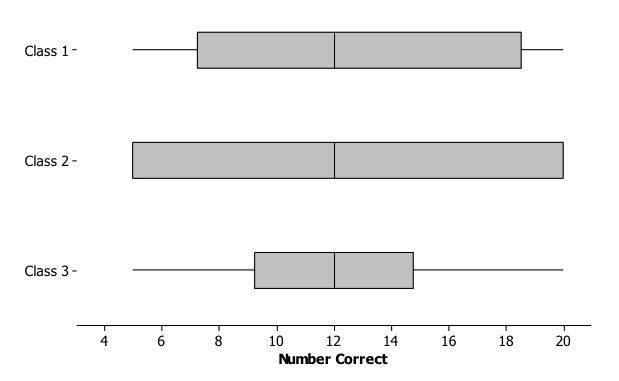# Descriptive Statistics

## Objective

Describe center and spread. Represent data in a box plot (box-and-whisker plot) and calculate the center and spread.

## Common Core Standards

### Core Standards

?

• HSS-ID.A.1 — Represent data with plots on the real number line (dot plots, histograms, and box plots).

• HSS-ID.A.2 — Use statistics appropriate to the shape of the data distribution to compare center (median, mean) and spread (interquartile range, standard deviation) of two or more different data sets.

?

• 6.SP.B.4

• 7.SP.B.4

## Criteria for Success

?

1. Understand that box plots are a way to organize univariate data, and identify the variable that the box plot is describing.
2. Describe center as a measurement of the middle of the data set. Median and mean are two measures of center.
3. Describe spread as how spread apart the data is in relation to the center. Interquartile range and standard deviation are the two measures of spread.
4. Create box plots using five number summaries consisting of the minimum, lower quartile, median, upper quartile, and maximum.
5. Identify the percentage of data that is located in each of the four quartiles, and analyze the box plot based on this information.
6. Describe that the middle 50% of the data is one measure of how spread out the data in the data set are. Define the range between the lower quartile and the upper quartile as the interquartile range.

## Tips for Teachers

?

• We are going to use what is known as the Moore and McCabe method for computing quartiles. This is where we DO NOT include the median in the calculation of the lower or upper quartiles.
• Excel uses Tukey’s method, which includes the median when calculating both the lower and upper quartile in a data set with an odd number of items.
• TI calculators use Moore and McCabe.
• Students will need to understand how to create box plots efficiently, so this lesson reviews material from 6th grade. However, there is not a lot of practice on creating box plots by hand, so if your students need practice in this, be sure to build it in.

## Anchor Problems

?

### Problem 1

Below is a dot plot of data showing the amount of time it takes students in Mr. S’s class to get to school.

• Draw a line separating the upper half of the data and the lower half of the data.
• Circle the median value.
• Draw a line showing half of the upper half of the data and another showing half of the lower half of the data.
• Estimate your box plot around the lines drawn in the dot plot.### Problem 2

The plot below shows the results of a survey of households about the number of dogs they have.
Identify the following statements as true or false. Explain your reasoning in each case.1. The maximum number of dogs per house is 8.
2. At least ${ \frac{1}{2} }$ of the houses have 2 or more dogs.
3. All of the houses have dogs.
4. Half of the houses surveyed have between 2 and 4 dogs.
5. Most of the houses surveyed have no dogs.

#### References

EngageNY Mathematics Grade 6 Mathematics > Module 6 > Topic C > Lesson 14Problem Set

Grade 6 Mathematics > Module 6 > Topic C > Lesson 14 of the New York State Common Core Mathematics Curriculum from EngageNY and Great Minds. © 2015 Great Minds. Licensed by EngageNY of the New York State Education Department under the CC BY-NC-SA 3.0 US license. Accessed Dec. 2, 2016, 5:15 p.m..

## Problem Set

?

The following resources include problems and activities aligned to the objective of the lesson that can be used to create your own problem set.

Include problems that require students to make comparisons between box plots, as well as create box plots from raw data sets or from five number summaries.

?

### Problem 1

Consider the following box plots, which show the number of questions different students in three different classes got correct on a 20-question quiz.• What is the interquartile range of class 3?
• What is the relationship between the median of each class data?
• What is the relationship between the interquartile range of each class data?
• How would you describe the comparison between the spread of data in each class?

#### References

EngageNY Mathematics Grade 6 Mathematics > Module 6 > Topic C > Lesson 15Exercise 11–15

Grade 6 Mathematics > Module 6 > Topic C > Lesson 15 of the New York State Common Core Mathematics Curriculum from EngageNY and Great Minds. © 2015 Great Minds. Licensed by EngageNY of the New York State Education Department under the CC BY-NC-SA 3.0 US license. Accessed Dec. 2, 2016, 5:15 p.m..

Modified by The Match Foundation, Inc.

### Problem 2

Given the following information, create a box plot and find the interquartile range.

For a large group of dogs, the shortest dog was 6 inches, and the tallest was 32 inches. One-half of the dogs were taller than 18 inches. One-fourth of the dogs were shorter than 15 inches. The upper quartile of the dog heights was 23 inches.

#### References

EngageNY Mathematics Grade 6 Mathematics > Module 6 > Topic C > Lesson 15Exit Ticket

Grade 6 Mathematics > Module 6 > Topic C > Lesson 15 of the New York State Common Core Mathematics Curriculum from EngageNY and Great Minds. © 2015 Great Minds. Licensed by EngageNY of the New York State Education Department under the CC BY-NC-SA 3.0 US license. Accessed Dec. 2, 2016, 5:15 p.m..